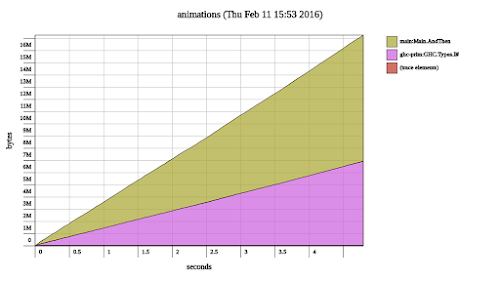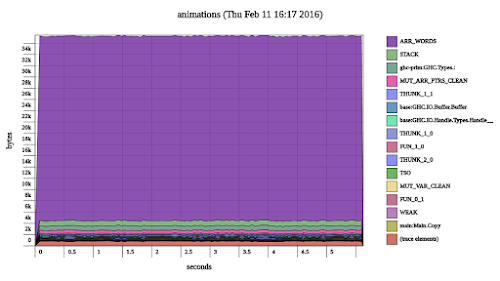## Monday, February 15, 2016

### Fixing a space leak by copying thunks.

After my last blog post about FRP I got feedback that my approach makes it easy to accidentally introduce space leaks. I also got helpful pointers to resources. In this post I give an example program with a space leak and discuss how to fix it.

### The `Behavior` type

We work with the following `Behavior` type:

``````data Behavior next a =
AndThen a (next (Behavior next a))

now :: Behavior next a -> a
now (AndThen a _) = a

future :: Behavior next a -> next (Behavior next a)
future (AndThen _ nextBehavior) = nextBehavior
``````

A behavior is a stream of values. It has a current value that we can extract with `now` and a future behavior that we can extract with `future`. The `Behavior` type constructor has two type parameters. The first type parameter called `next` lets us decide which effects we want to allow when we advance to the next iteration. The second type parameter called `a` is the type of values this behavior carries. The behavior type is the same (up to renaming) as `Cofree`.

We can instantiate `next` with different types. We have instances of `Functor` and `Applicative` for `Behavior` as long as we instantiate `next` with types that have instances of `Functor` and `Applicative`:

``````instance (Functor next) => Functor (Behavior next) where

fmap f (a `AndThen` nextAs) =
(f a) `AndThen` (fmap (fmap f) nextAs)

instance (Applicative next) => Applicative (Behavior next) where

pure a = a `AndThen` (pure (pure a))

(f `AndThen` nextFs) <*> (x `AndThen` nextXs) =
(f x) `AndThen` (liftA2 (<*>) nextFs nextXs)
``````

Just a note: this `Applicative` instance is different to the one for `Cofree`.

In the last post `next` was `IO` which allows arbitrary side-effects and is hard to reason about. But we can for example instantiate `next` to `Identity` which means we get ordinary pure lists. Because behaviors over `Identity` are like lists we have correspondences between `fmap` and `map`, `now` and `head` and `liftA2` and `zipWith`.

To test behaviors where `next` is `Identity` we use a function that writes its elements to the console:

``````runList :: (Show a) => Behavior Identity a -> IO ()
runList (a `AndThen` as) = do
print a
runList (runIdentity as)
``````

We use `runIdentity` to get the next behavior to run.

### A leaky program

Let’s create a program with a space leak! We will take inspiration from programs on lists. We use two functions that correspond to `enumFrom` and `repeat` called `countFrom` and `always`. `countFrom` takes an `Int` and starts counting from that number. `always` lets us transport any value indefinitely far into the future. They work for behaviors with all sorts of instantiations for `next` as long as they are `Applicative` because they use `pure`.

``````countFrom :: (Applicative next) => Int -> Behavior next Int
countFrom i = i `AndThen` pure (countFrom (i + 1))

always :: (Applicative next) => a -> Behavior next a
always a = a `AndThen` pure (always a)
``````

We will use `countFrom` to create a behavior called `numbers`. We will pair it with a behavior that is always zero. When we run the final program (for historical reasons named `animations`) the output looks like this:

``````> animations
(0,0)
(1,0)
(2,0)
(3,0)
...
``````

The trick is that the second element of the printed pairs will require a reference to `numbers`. This means that `numbers` cannot be garbage collected. As `numbers` expands it will take up more and more heap space. The program looks like this:

``````main :: IO ()
main = do

let numbers = countFrom 0

let alwaysNumbers = fmap now (always numbers)

let pairs = liftA2 (,) numbers alwaysNumbers

runList pairs
``````

We transport `numbers` into the future using `always`. This would be a behavior of behaviors. At each point in time we extract the current value to get a behavior of `Int` using `fmap now`. We call the resulting behavior `alwaysNumbers`. Then we zip `numbers` and `alwaysNumbers` together using `liftA2`. Finally we run the behavior (which means to keep iterating and printing).

Here is a heap profile created by invoking the program with `animations +RTS -h`. We invoked `hp2pretty` on `animations.hp` to produce the following picture:Space usage grows linearly with time.

### The problem pictorially

The following shows a sketch of the heap after the program printed `(3,0)`:The chain of cells represents the `numbers` value. The two arrows coming from the top are two references: one to the original `numbers` value and one to the current element. The little cloud represents the unevaluated thunk that is the future behavior.

Now, what if we didn’t have a single chain of cells? What if we copied all future behavior thunks and did not replace them? We would get a picture like this:The garbage collector will reclaim the cells for `1` and `2` because there are no references to them.

### Copying thunks

Let me demonstrate what I mean with “copying thunks” with a small `ghci` session. On a high level we bind `exampleThunk` to the pure value `True` and evaluate it three times. To observe the evaluation we use `trace` from `Debug.Trace` to print `"thunk evaluated"` upon evaluation.

``````> import Debug.Trace
> let exampleThunk = trace "thunk evaluated" True
> exampleThunk
thunk evaluated
True
> exampleThunk
True
> exampleThunk
True
``````

The string `"thunk evaluated"` appears only once. Later evaluations reuse the previously computed value. Usually this is a good thing because the thunk might have been costly to evaluate.

But now what we’d like to have are two functions with the following signatures:

``````copy :: a -> Copy a
getCopy :: Copy a -> a
``````

This time we use functions `copy` and `getCopy` to make the evaluation of `exampleThunk` happen multiple times.

``````> import Debug.Trace
> let exampleThunk = trace "thunk evaluated" True
> let copyOfExampleThunk = copy exampleThunk
> getCopy copyOfExampleThunk
thunk evaluated
True
> getCopy copyOfExampleThunk
thunk evaluated
True
> getCopy copyOfExampleThunk
thunk evaluated
True
``````

Whenever we force the result of `getCopy` the thunk is evaluated. We do not replace the original thunk by the result.

### The `Copy` Applicative

I don’t think it is possible to get the desired behavior of `Copy` with current GHC. But luckily Joachim Breitner has published an experiment in the form of the `ghc-dup` package a few years ago. It works (as a prototype) for GHC 7.4 and GHC 7.6. It provides a function `dup` that allows us to create the desired `Copy` type and make it an `Applicative`:

``````data Copy a = Copy a

copy :: a -> Copy a
copy a = Copy a

getCopy :: Copy a -> a
getCopy (Copy a) = case dup a of Box a' -> a'

instance Functor Copy where
fmap f a = copy (f (getCopy a))

instance Applicative Copy where
pure a = copy a
f <*> a = copy ((getCopy f) (getCopy a))
``````

We are careful to never directly use the thunk inside `Copy`. Instead we copy it with `getCopy`.

### Heap profile with copying

Now we can instantiate our behavior with `Copy` instead of with `Identity` and still run it like so:

``````runCopying :: (Show a) => Behavior Copy a -> IO ()
runCopying (a `AndThen` copyAs) = do
print a
runCopying (getCopy copyAs)
``````

We use `getCopy` instead of `runIdentity` to get the next behavior. This allows us to change the previously leaky program to use `Copy` instead of `Identity` by using `runCopying` instead of `runList`:

``````main :: IO ()
main = do

let numbers = countFrom 0

let alwaysNumbers = fmap now (always numbers)

let pairs = liftA2 (,) numbers alwaysNumbers

runCopying pairs
``````

We get the same output but the following heap profile:The space leak is gone.

### Conclusion

GHC uses call-by-need by default. It allows for call-by-value via `seq` and `deepseq`. Sometimes we want call-by-name. I believe that if `ghc-dup` was a feature supported by GHC we would see it used.